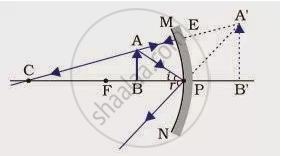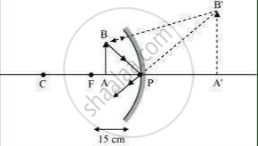# We Wish to Obtain an Erect Image of an Object, Using a Concave Mirror of Focal Length 15 Cm. What Should Be the Range of Distance of the Object from the Mirror? - Science

We wish to obtain an erect image of an object, using a concave mirror of focal length 15 cm. What should be the range of distance of the object from the mirror? What is the nature of the image? Is the image larger or smaller than the object? Draw a ray diagram to show the image formation in this case.

#### Solution 1

Range of the distance of the object = 0 to 15 cm from the pole of the mirror.
Nature of the image = virtual, erect and larger than the object.#### Solution 2

Range of object distance = 0 cm to15 cm
A concave mirror gives an erect image when an object is placed between its pole (P) and the principal focus (F).
Hence, to obtain an erect image of an object from a concave mirror of focal length 15 cm, the object must be placed anywhere between the pole and the focus. The image formed will be virtual, erect, and magnified in nature, as shown in the given figure.Concept: Concave Mirror
Is there an error in this question or solution?
Chapter 10: Light – Reflection and Refraction - Exercises [Page 186]

#### APPEARS IN

NCERT Class 10 Science
Chapter 10 Light – Reflection and Refraction
Exercises | Q 7 | Page 186

Share